# Banach lattice

A vector lattice that is at the same time a Banach space with a norm which satisfies the monotonicity condition:A Banach lattice is also called a KB-lineal, whereas an arbitrary normed lattice, i.e. a vector lattice with a monotone norm, is called a KN-lineal. When completing a normed lattice in norm, the order relations may be extended to the resulting Banach space so that it becomes a Banach lattice. If it is possible to introduce in a lattice a Banach topology which converts it to a Banach lattice, such a topology is unique. The simplest example of a Banach lattice is the space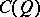of continuous functions on an arbitrary compact topological space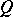with the natural (pointwise) order and with the ordinary (uniform) norm. Other examples of Banach lattices include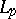spaces and Orlicz spaces (cf. Orlicz space). In Banach lattices convergence in norm is ()-convergence for convergence with a regulator. This is not true of normed lattices.

An important special case is a Banach lattice of bounded elements. If a latticecontains a strong unit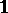, i.e. if for eachthere exists asuch that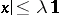, then the smallestfor which this inequality is valid is taken as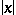. The normed lattice thus obtained is called a normed lattice of bounded elements; if it is complete in norm, it is called a Banach lattice of bounded elements. In a Banach lattice (and even in a normed lattice) of bounded elements convergence in norm is identical with convergence with a regulator, while the boundedness of a set of elements in norm is identical with order boundedness. If a normed lattice of bounded elements is conditionally-complete, it is complete in norm.

The spaceis a Banach lattice of bounded elements in which the function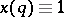is taken as the unit. For any Banach latticeof bounded elements there exists a compact Hausdorff spacesuch thatis algebraically and lattice isomorphic to the space. This is an abstract characterization of the Banach lattice of continuous functions on a compact Hausdorff space.

In any normed lattice an additive functional that is continuous in norm is regular and, moreover, is representable as the difference of two additive functionals which are continuous in norm. In a Banach lattice each positive additive functional is continuous in norm, which means that the classes of regular functionals and additive functionals which are continuous in norm coincide. The spacewhich is dual in the sense of Banach to the normed latticeis a conditionally complete Banach lattice. In a normed lattice the Hahn–Banach theorem may be strengthened as follows: For anythere exists a positive additive functional, which is continuous in norm, such that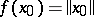,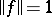.

How to Cite This Entry:
Banach lattice. B.Z. Vulikh (originator), Encyclopedia of Mathematics. URL: http://encyclopediaofmath.org/index.php?title=Banach_lattice&oldid=15867
This text originally appeared in Encyclopedia of Mathematics - ISBN 1402006098